Ex 9.1

Chapter 9 Class 10 Some Applications of Trigonometry
Serial order wise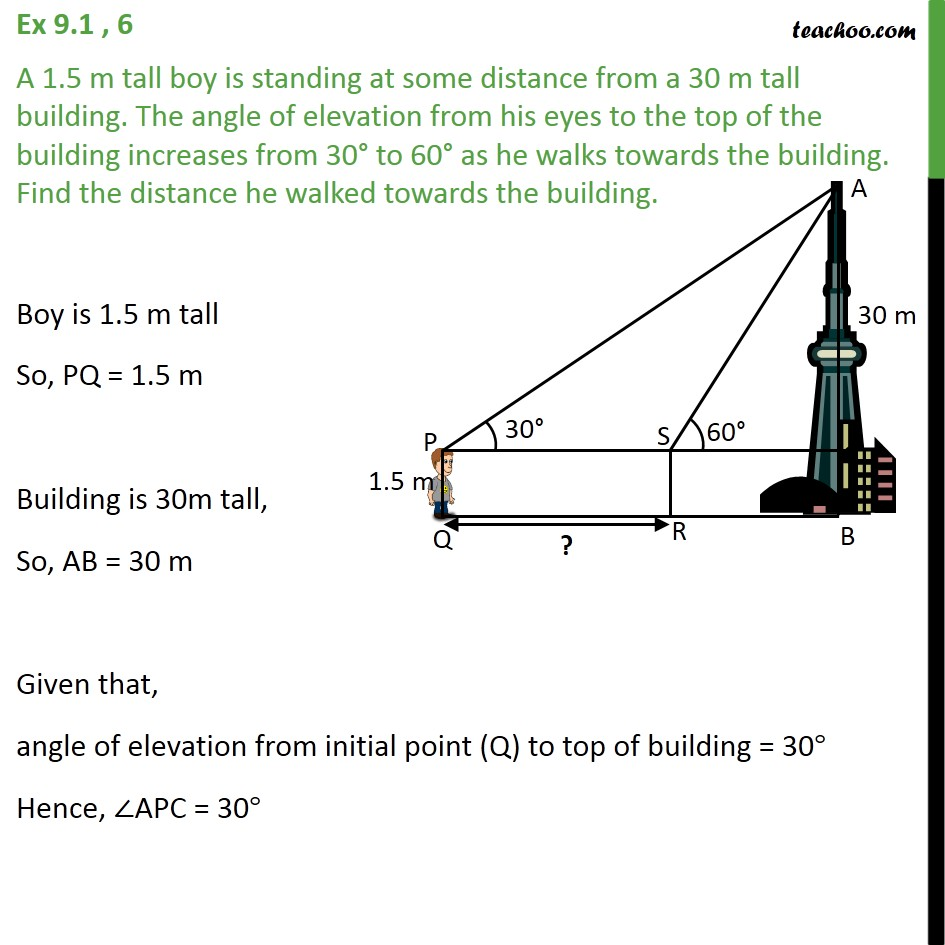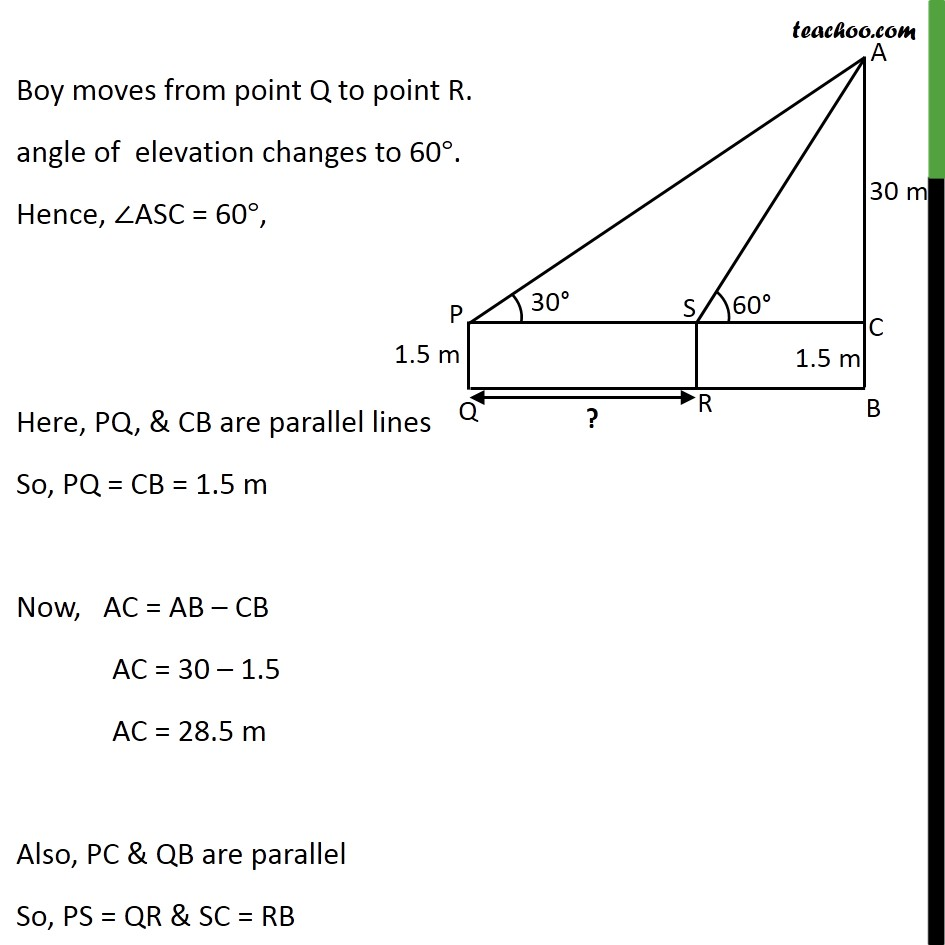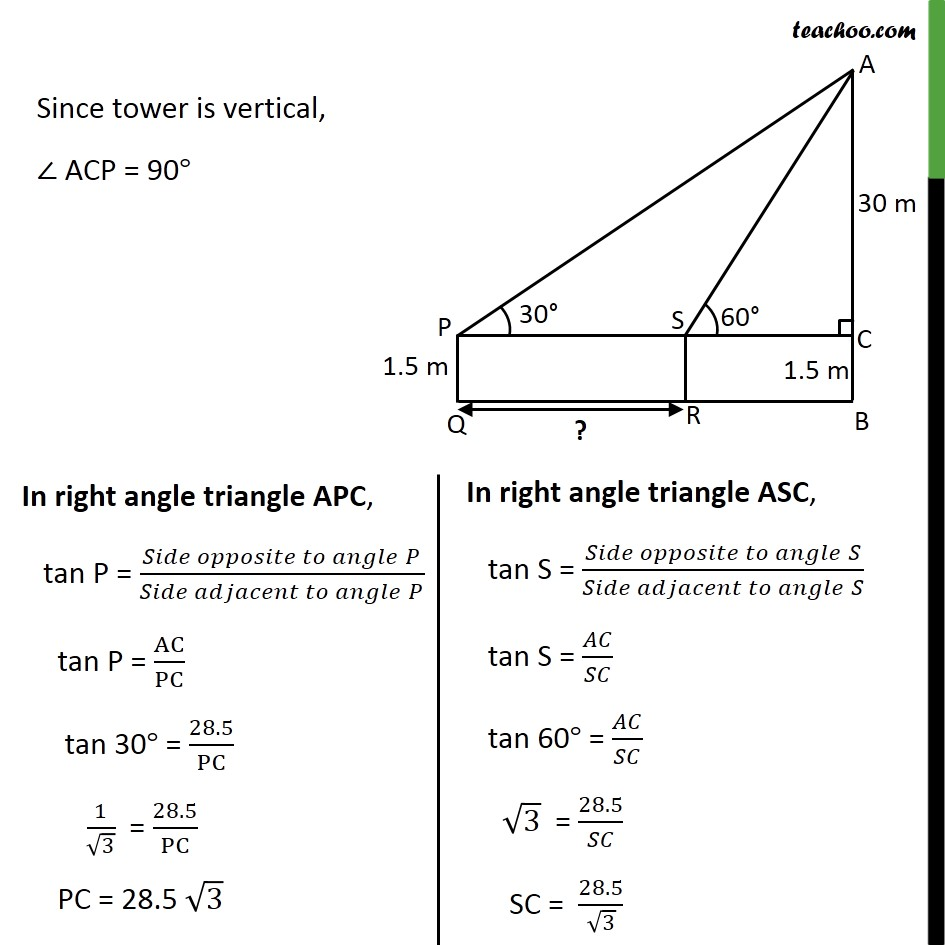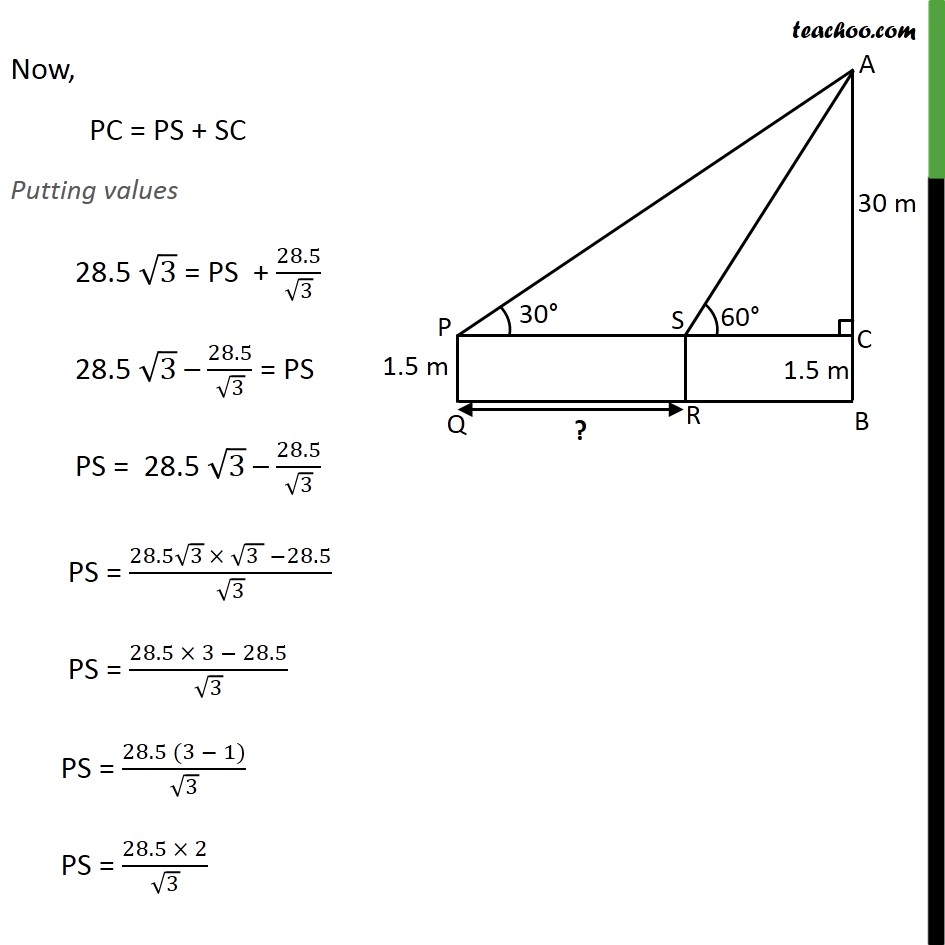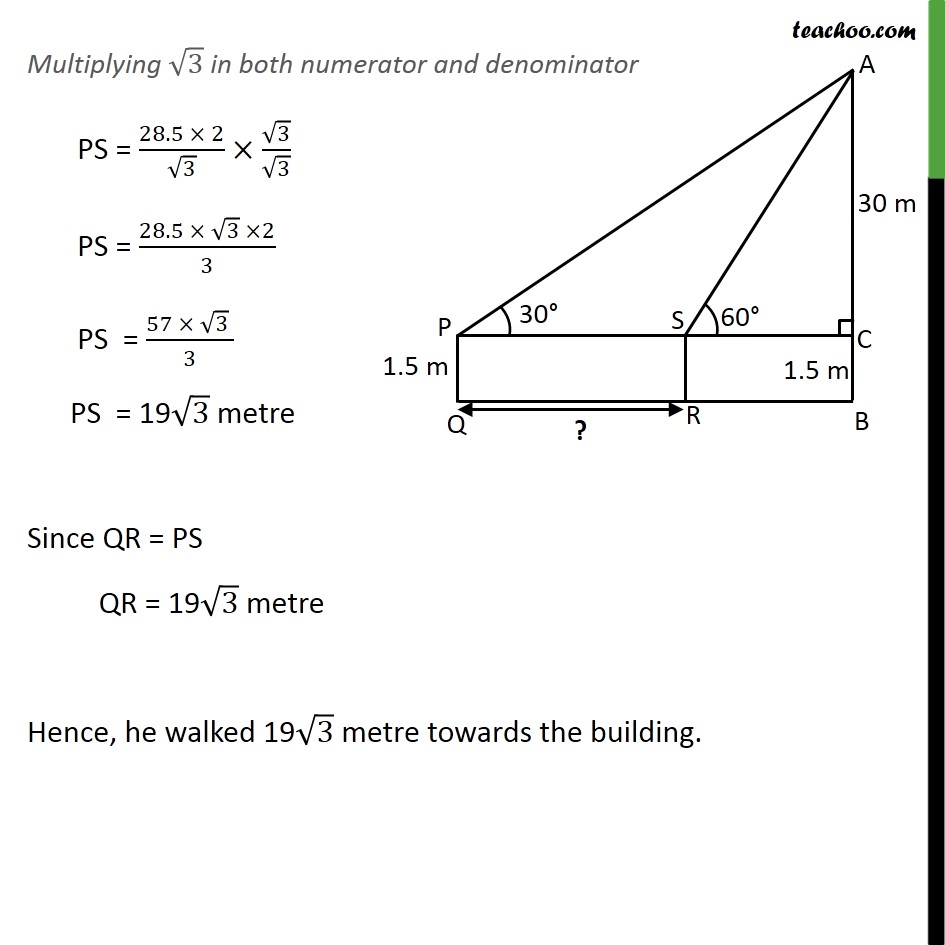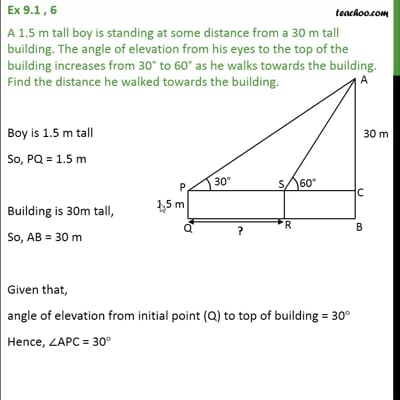This video is only available for Teachoo black users

Solve all your doubts with Teachoo Black (new monthly pack available now!)

### Transcript

Ex 9.1 , 6 A 1.5 m tall boy is standing at some distance from a 30 m tall building. The angle of elevation from his eyes to the top of the building increases from 30 to 60 as he walks towards the building. Find the distance he walked towards the building. Boy is 1.5 m tall So, PQ = 1.5 m Building is 30m tall, So, AB = 30 m Given that, angle of elevation from initial point (Q) to top of building = 30 Hence, APC = 30 Boy moves from point Q to point R. angle of elevation changes to 60 . Hence, ASC = 60 , Here, PQ, & CB are parallel lines So, PQ = CB = 1.5 m Now, AC = AB CB AC = 30 1.5 AC = 28.5 m Also, PC & QB are parallel So, PS = QR & SC = RB Since tower is vertical, ACP = 90 Now, PC = PS + SC Putting values 28.5 3 = PS + 28.5/ 3 28.5 3 28.5/ 3 = PS PS = 28.5 3 28.5/ 3 PS = (28.5 3 (3 ) 28.5)/ 3 PS = (28.5 3 28.5)/ 3 PS = (28.5 (3 1))/ 3 PS = (28.5 2)/ 3. Multiplying 3 in both numerator and denominator PS = (28.5 2)/ 3 3/ 3 PS = (28.5 3 2)/3 PS = (57 3 )/3 PS = 19 3 metre Since QR = PS QR = 19 3 metre Hence, he walked 19 3 metre towards the building.